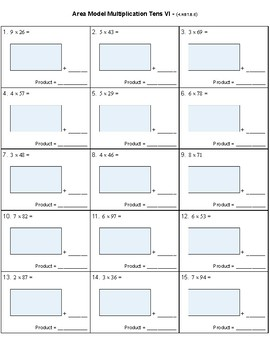# Area Model Multiplication WorksheetsSubject
Resource Type
File Type

PDF

(6 MB|58 pages)
Product Description

Area Model Multiplication Worksheets

-Instructions on how to do Area Models.

-Times Tables - 50 mixed multiplication problems at different levels to prepare.

-Multiplication Strategies - Decompose using distributive property to make harder math facts easier. Ex. 6x9 = (3x9) + (3x9).

-Third grade: area models for math facts and for tens.

-4th grade: area models for two-digit x single, three-digit x single, four-digit x single, and two-digit x two-digit problems.

-5th grade area models for two-digit x three digit, three-digit x three digit, and multiplying decimals.

58 pages of easy, medium, and hard problems.

Common Core Standards covered:

3.OA.B.5

4.OA.B.4

4.NBT.B.5

5.NBT.B.5

5.NBT.B.7

Total Pages
58 pages
Not Included
Teaching Duration
N/A
Report this Resource to TpT
Reported resources will be reviewed by our team. Report this resource to let us know if this resource violates TpT’s content guidelines.